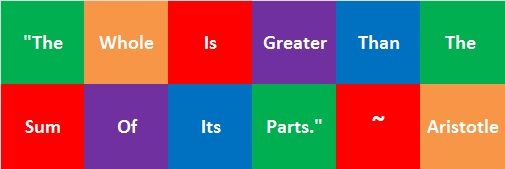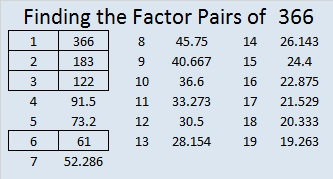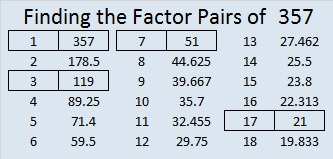# 508 A Mathematical and Biblical Truth: 2 > 1Two are better than one; because they have a good reward for their labour.

For if they fall, the one will lift up his fellow: but woe to him that is alone when he falleth; for he hath not another to help him up.

Ecclesiastes 4: 9 – 10

—————————————————————————————————

508 is the sum of some consecutive prime numbers, and at least one of those prime numbers is in its prime factorization. Can you figure out what those consecutive primes are?

• 508 is a composite number.
• Prime factorization: 508 = 2 x 2 x 127, which can be written 508 = (2^2) x 127
• The exponents in the prime factorization are 2 and 1. Adding one to each and multiplying we get (2 + 1)(1 + 1) = 3 x 2  = 6. Therefore 508 has exactly 6 factors.
• Factors of 508: 1, 2, 4, 127, 254, 508
• Factor pairs: 508 = 1 x 508, 2 x 254, or 4 x 127
• Taking the factor pair with the largest square number factor, we get √508 = (√4)(√127) = 2√127 ≈ 22.53 88 55 33 9# 440 Can You Appreciate a Good Paradox?

Brainden.com gives several examples of paradoxes as well as this definition: “A paradox is a statement that contradicts itself or a situation which seems to defy logic.”

Alan Parr suggested I write no more than the fact that 440 is the sum of consecutive prime numbers. It may seem to defy logic that he would want to try to figure out what those consecutive primes are without any hints, but he proved yesterday he is up for the challenge. Check the comments to see if he (or somebody else) can figure out what those prime numbers might be. I am waiting to see if he can again defy logic and figure them out. In the meantime, the factors of 440 will be listed at the end of this post.

I remember watching the episode of Star Trek in which several androids malfunctioned because they couldn’t handle paradoxes, but most humans seem to be able to handle paradoxes just fine. I’ve written one myself that I hope you will enjoy:

I overheard a pair of docs in the middle of a heated argument. One of them said, “As I have stated hundreds of times before, no matter what I say, YOU ALWAYS contradict me!” The other doc shook his head saying, “No, no. That’s not correct at all. YOU’RE the one who’s always contradicting ME!” I hate to hear arguing, so I left, but I have to wonder if they ever worked it out or are they still arguing about contradicting each other even today?

You might not expect to find paradoxes in the The New Testament, but crosswalkblogdotcom describes several of them beautifully in I-will-take-paradox-for-200-alec.

Shakespeare and Wordsworth used them as well. See examples in Is-this-phrase-grammatically-and-semantically-correct?

When I first joined twitter, this is part of the first conversation I had:

Steve Morris has written about paradoxes on several occasions including his very popular post titled Failure-to-fail. If we fail to fail, we become the biggest failure.

It is a paradox to have to be willing to fail in order to really succeed. As Michael Jordan stated in a Nike commercial: “I’ve missed more than 9000 shots in my career. I’ve lost almost 300 games. 26 times, I’ve been trusted to take the game winning shot and missed. I’ve failed over and over and over again in my life. And that is why I succeed.”

We will face setbacks, trials of faith, and other paradoxes in our lives, but we must not give up. All of them are opportunities for us to learn and grow. We actually ought to be grateful for them, because as we face them, we become stronger and more successful. Zeno’s paradox would have us believe that we can never reach our goals. That is simply not true. We can reach them if we keep them in our sights and keep going toward them relentlessly.

Here are the factors of 440:

• 440 is a composite number.
• Prime factorization: 440 = 2 x 2 x 2 x 5 x 11, which can be written 440 = (2^3) x 5 x 11
• The exponents in the prime factorization are 3, 1, and 1. Adding one to each and multiplying we get (3 + 1)(1 + 1)(1 + 1) = 4 x 2 x 2 = 16. Therefore 440 has exactly 16 factors.
• Factors of 440: 1, 2, 4, 5, 8, 10, 11, 20, 22, 40, 44, 55, 88, 110, 220, 440
• Factor pairs: 440 = 1 x 440, 2 x 220, 4 x 110, 5 x 88, 8 x 55, 10 x 44, 11 x 40, or 20 x 22
• Taking the factor pair with the largest square number factor, we get √440 = (√4)(√110) = 2√110 ≈ 20.9762Since 440 = 20 × 22, we know that 441 = 21 × 21.

# 433 The Whole Is Greater Than the Sum of Its Parts

Today I practiced a song with a choir comprised of nine smaller choirs. Every time before I had only practiced the song with our smaller choir. As I listened to all the voices in this big choir while we sang in harmony, I thought, “A big choir sounds SO good. The whole is greater than the sum of its parts!”Here is a professional recording of the beautiful song we are singing. The music in this youtube video is directed by the composer, Rob Gardner. It features the London Symphony Orchestra at Air Studios, London and the Spire Choir at Camelback Baptist Church in Paradise Valley, Arizona.

The number 433 is also greater than the sum of its parts.

12² + 17² = 433

A Chinese checker board looks like a star and has 121 holes so 121 is called a star number. The first star number is 1, followed by 13, 37, 73, and 121. The ninth star number is 433.

433 is one more than twelve times the eighth triangular number. Star numbers and triangular numbers are related like that.

145-408-433 is a primitive Pythagorean triple.

Here is the factoring information for the number 433:

• 433 is a prime number. 431 and 433 are twin primes.
• Prime factorization: 433 is prime.
• The exponent of prime number 433 is 1. Adding 1 to that exponent we get (1 + 1) = 2. Therefore 433 has exactly 2 factors.
• Factors of 433: 1, 433
• Factor pairs: 433 = 1 x 433
• 433 has no square factors that allow its square root to be simplified. √433 ≈ 20.8087How do we know that 433 is a prime number? If 433 were not a prime number, then it would be divisible by at least one prime number less than or equal to √433 ≈ 20.8087. Since 433 cannot be divided evenly by 2, 3, 5, 7, 11, 13, 17, or 19, we know that 433 is a prime number.

# A Teacher Affects Eternity and Divisibility Tricks Applied to 418

Henry Brooks Adams said, “A teacher affects eternity; he can never tell where his influence stops.” Of course the quote applies to female teachers just as much as it does to male teachers. Affecting eternity is pretty serious business.

It will probably take less than two minutes to read Marek Bennet’s  comic on how the work you do fits into the educational system. Its message is worth pondering for quite a long time.Let me demonstrate some quick prime number divisibility tricks on the number 418:

• It’s even so its divisible by 2.
• 4 + 1 + 8 = 13, which is not a multiple of 3, so 418 is not divisible by 3.
• The last digit isn’t 0 or 5, so 418 is not divisible by 5.
• 41 – 2(8) = 41 – 16 = 25, which is not a multiple of 7, so 418 is not divisible by 7.
• If we add the first and the 3rd digits of 418, we get twelve. Then if we subtract the second digit, one, we get eleven. Since we got a multiple of eleven, that means that our original number, 418, can be evenly divided by eleven.

Here is the factoring information for 418:

• 418 is a composite number.
• Prime factorization: 418 = 2 x 11 x 19
• The exponents in the prime factorization are 1, 1, and 1. Adding one to each and multiplying we get (1 + 1)(1 + 1)(1 + 1) = 2 x 2 x 2 = 8. Therefore 418 has exactly 8 factors.
• Factors of 418: 1, 2, 11, 19, 22, 38, 209, 418
• Factor pairs: 418 = 1 x 418, 2 x 209, 11 x 38, or 19 x 22
• 418 has no square factors that allow its square root to be simplified. √418 ≈ 20.445# 393 and A Chance to Solve a ProblemA few weeks ago Brilliant Quotes tweeted a quote from Duke Ellington: “A problem is a chance for you to do your best.”

I immediately thought of problems students are given in mathematics classes because this quote applies to those problems.

It also applies to any other kind of problem a person may face. Sometimes those problems are much more challenging than problems involving numbers. All problems are opportunities for us to do our best.

Let’s find the factoring information for a relatively easy number – 393.

• 393 is a composite number.
• Prime factorization: 393 = 3 x 131
• The exponents in the prime factorization are 1 and 1. Adding one to each and multiplying we get (1 + 1)(1 + 1) = 2 x 2 = 4. Therefore 393 has exactly 4 factors.
• Factors of 393: 1, 3, 131, 393
• Factor pairs: 393 = 1 x 393 or 3 x 131
• 393 has no square factors that allow its square root to be simplified. √393 ≈ 19.824Is 393 in any Pythagorean triples?

• 393-524-655  which is [3 – 4 – 5] times 131
• 393-25740-25743 which is [131-8580-8581] times 3
• Primitive 393-8576-8585
• and Primitive 393-77224-77225

At the end of his post a-promise-broken, Established1962 tells a personal and very funny story involving his copy of Duke Ellington’s autobiography, “Music Is My Mistress.” The quote above is included in that book.

# 366 and a Quote from Euclid

The laws of nature are but the mathematical thoughts of God.  ~Euclid

Euclid was a Greek mathematician who was born about 300 BC. I realize when he wrote these words (in ancient Greek), they didn’t mean the exact same thing to him that they mean to me. Nevertheless, I like the quote very much, and find infinite beauty in its message. The laws of nature are but the mathematical thoughts of God.  ~Euclid

Since writing this post, I have found some other lovely representations of this quote on twitter:

It is well worth pushing the button to see this next gif in action:

Here are some mathematical thoughts about the number 366:

• 366 is a composite number.
• Prime factorization: 366 = 2 x 3 x 61
• The exponents in the prime factorization are 1, 1, and 1. Adding one to each and multiplying we get (1 + 1)(1 + 1)(1 + 1) = 2 x 2 x 2 = 8. Therefore 366 has exactly 8 factors.
• Factors of 366: 1, 2, 3, 6, 61, 122, 183, 366
• Factor pairs: 366 = 1 x 366, 2 x 183, 3 x 122, or 6 x 61
• 366 has no square factors that allow its square root to be simplified. √366 ≈ 19.13166² + 360² = 366² How do I know? Because 11² + 60² = 61² so (6·11)² + (6·60)² = (6·61)².

366 is a palindrome in a couple of bases:

• 303 in BASE 11 because 3(11²) + 0(11¹) + 3(11º) = 366
• 222 in BASE 13 because 2(13²) + 2(13¹) + 2(13º) = 366

These are just a few of the things known by the Greatest Mathematician of All! He completely understands EVERYTHING from infinitesimal to infinity. By His grace I understand a tiny bit of what He knows.

# 357 Equality, Mathematics, and Dr. Martin Luther King, Jr.

I read several quotes from Dr. Martin Luther Kings, Jr. today, including this one on equality and integration. The last sentence mentions mathematics, but I’m not exactly sure what that last sentence has to do with the rest of the quotation. Can anyone enlighten me? (Check the comments for one interpretation.)Here is a little about the number 357:

3, 5, and 7 are three consecutive odd numbers so, so 357 is divisible by 3 and is a composite number.

• 357 is a composite number.
• Prime factorization: 357 = 3 x 7 x 17
• The exponents in the prime factorization are 1, 1, and 1. Adding one to each and multiplying we get (1 + 1)(1 + 1)(1 + 1) = 2 x 2 x 2 = 8. Therefore 357 has exactly 8 factors.
• Factors of 357: 1, 3, 7, 17, 21, 51, 119, 357
• Factor pairs: 357 = 1 x 357, 3 x 119, 7 x 51, or 17 x 21
• 357 has no square factors that allow its square root to be simplified. √357 ≈ 18.894